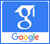Magnetohydrodynamic equationsMagnetohydrodynamic (MHD) stability of wendelstein7-X reactor with resistive wall (RWMs)

Published on: 22nd May, 2021

Plasma stability is the biggest challenge facing the nuclear fusion industry. One of the best methods of stability study is magnetohydrodynamic (MHD) equations, which has two linear and nonlinear states. Usually linear stability analysis is used to describe the MHD state, which is obtained by linearizing nonlinear equations. The reactor under study is the W7-X reactor, which is an optimal example of a stellaratoric system. The question raised in this research is how to create suitable conditions for the formation of plasma and heat transfer produced by the melting reaction. Many efforts have been made in this direction, but still the record holder for plasma state maintenance belongs to the international ITER project and around 1000. However, IPP researchers at the Max Planck Institute in Germany (maker of the W7-X reactor) predicted that by 2020 they would produce a pulse of 30 minutes. The numerical method is used to investigate the stability of the reactor. In this paper, boundary conditions were expressed in terms of resistance wall. With the help of the mathematical Matlab software, magnetic field values ​​were obtained from experimental reports extracted from the Max Planck Institute for various values ​​of β. From the values ​​obtained, it was concluded that the appropriate field value is β = 5 according to the ideal MagnetoHydroDynamic state and the interval defined by the Max Planck Institute.